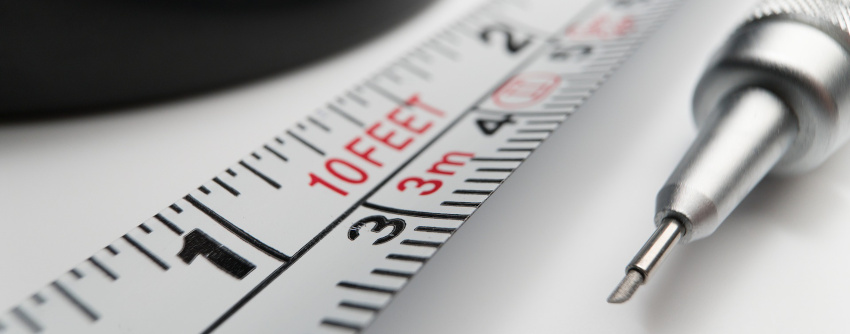# Unit Converters

Share:Here we have several unit converters and calculators used for converting measurement units from Metric SI system to US Customary system and vice versa. This collection of conversion calculators is growing. Currently we have calculators for distance and length measurement units, weight and mass units, temperature units, volume measurement units and even converters for kitchen and cooking measuring units.

To go to the calculator, find and press the icons below. It will take you the designated unit conversion calculator. In the calculator page, you will have the provision to enter the units and on pressing the convert button, the results will be displayed.

Each metric conversion calculator comes with a chart. You can customize and generate the chart with your inputs like starting units, increments and print them. The conversion charts even come up with options to switch the from and to measurement units and option for rounding the decimal values.

These calculators are designed with user friendliness and precision in mind. You can get results for up to 8 decimal places. Even the chart can be generated with up to 8 decimal places. Try the calculators and generate your own charts.

## Distance And Length Conversion Calculators

These distance and length conversion calculators helps you to convert Metric SI Units of Length like meter (m), centimeter (cm), kilometer (km), etc. to US customary (inch, foot, mile, etc.), US survey and US nautical units and vice versa.

## Weight And Mass Unit Converters

The mass and weight calculators converts numeric values between Metric SI System units like kilogram (kg), gram (g), milligram (mg), etc. and Metric Non-SI System units like metric tonne and metric kilotonne to other unit systems like US Customary Mass units and vice versa.

## Temperature Metric Converters

This calculator converts temperature units like Fahrenheit to Celsius and vice versa.

## Cooking And Kitchen Measuring Unit Converters

Calculators and charts to convert measuring units used for kitchen and cooking. This converter uses density of the food item to convert between volume and weight and vice versa.

## Volume Unit Converters

Calculators to convert Metric SI volume units like litre (l), milliliter (l), etc. to US customary volume units like cup, fluid ounce, pint, etc.. You can even convert them to British imperial volume units.